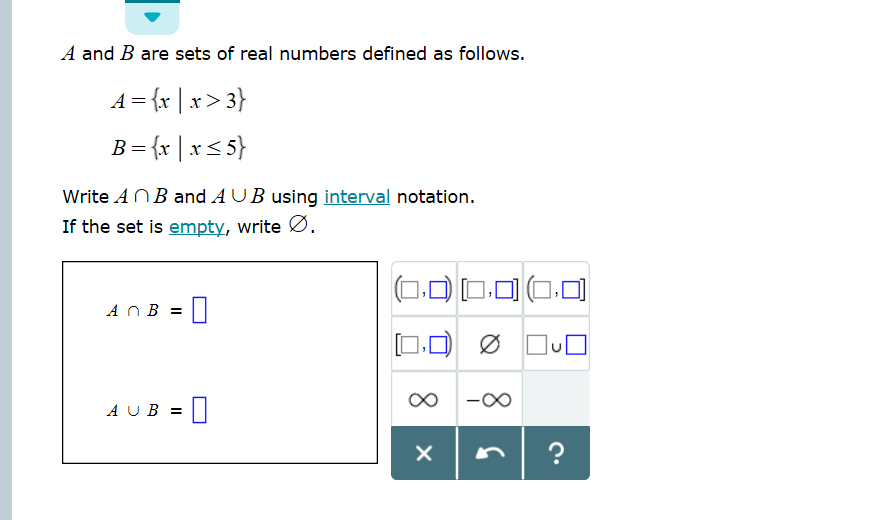# A and B are sets of real numbers defined as follows. 4 = {x | x > 3} Bx|x5 Write A n B and A UB using interval notation. If the set is empty, write Ø. -oO AU B X Il

Questionhelp_outlineImage TranscriptioncloseA and B are sets of real numbers defined as follows. 4 = {x | x > 3} Bx|x5 Write A n B and A UB using interval notation. If the set is empty, write Ø. -oO AU B X Il fullscreen

### Want to see this answer and more?

Experts are waiting 24/7 to provide step-by-step solutions in as fast as 30 minutes!*